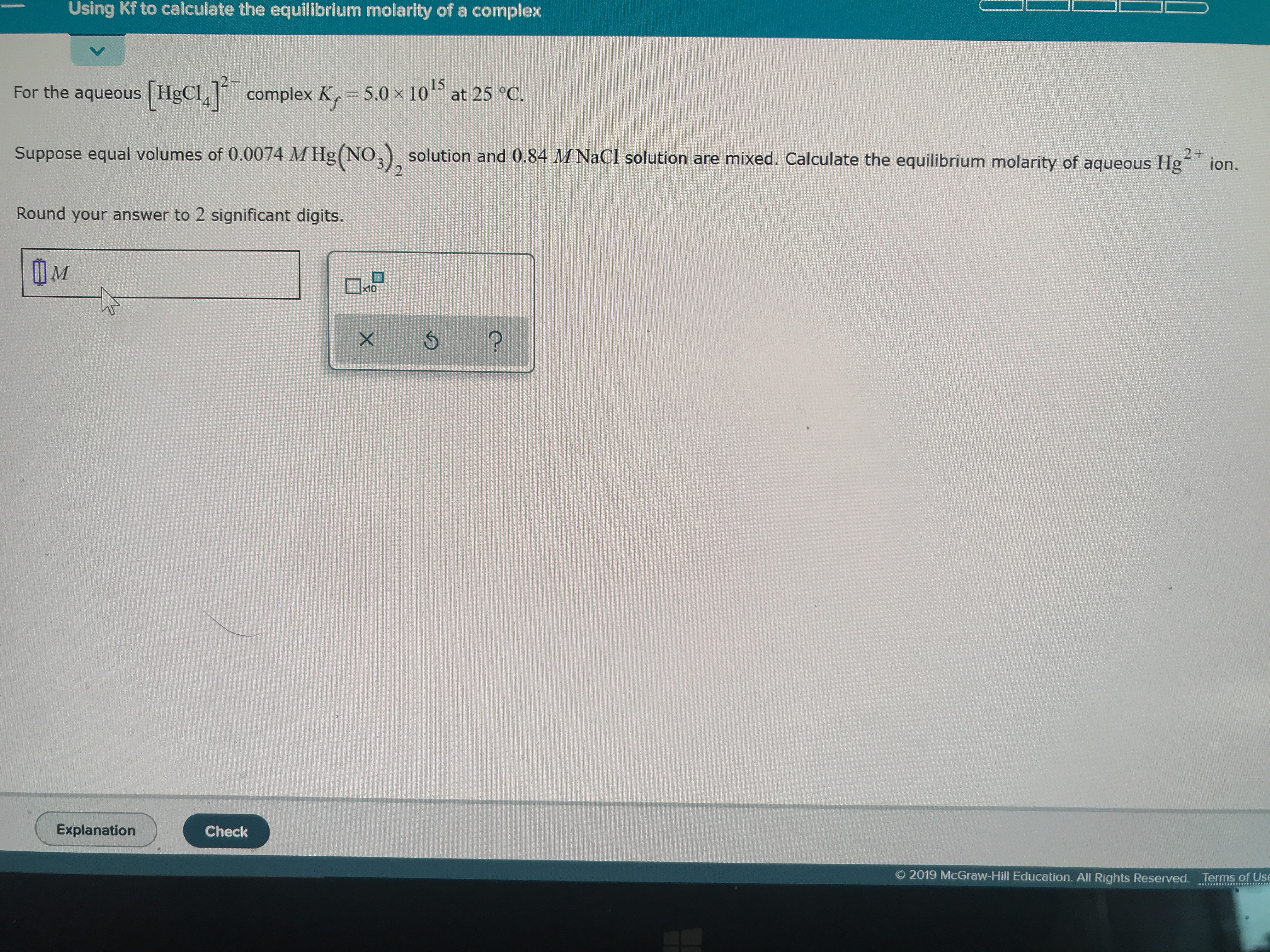# For the aqueous [HgCl4]2- complex Kf=5.0x10^15 at 25C. Suppose equal volumes of 0.0074 M Hg(NO3)2 solution and 0.84 M NaCl solution are mixed. Calculate the equilibrium molarity of aqueous Hg2+. Round to two significant digits